# Gauge group acts on affine space of connections

## Statement

Suppose$M$ is a differential manifold and$E$ is a vector bundle over$M$. We define the gauge group$G$ of$E$ as the group of all smooth maps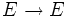$E \to E$ that sends the fiber over any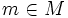$m \in M$ to itself, and is a linear automorphism for every such fiber.

The gauge group acts on the affine space of connections of$M$. Here, we describe this action in three ways, using the three alternative descriptions of a connection.

### Action with respect to the usual definition of connection

In the usual definition, a connection is defined globally, as a map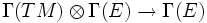$\Gamma(TM) \otimes \Gamma(E) \to \Gamma(E)$, satisfying certain conditions. Suppose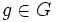$g \in G$ and$\nabla$ is a connection on$M$. We define the connection$g . \nabla$ as follows: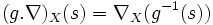$(g . \nabla)_X(s) = \nabla_X(g^{-1}(s))$

The inverse sign comes to preserve the left action condition.

With this definition, it is fairly easy to see that the new map is again a connection, and moreover, the action of any$g \in G$ is a linear map, and in particular, an affine map.

### Action with respect to the view of a connection as a module structure

Further information: Connection is module structure over connection algebra

A connection on a vector bundle$E$ over$M$ can be viewed as equipping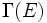$\Gamma(E)$ with the structure of a module over the connection algebra of$M$. Let us understand how an element$g \in G$ acts on$E$.

A connection$\nabla$ is viewed in terms of its action map: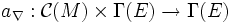$a_\nabla:\mathcal{C}(M) \times \Gamma(E) \to \Gamma(E)$

The action map of$g.\nabla$ is given by: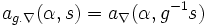$a_{g.\nabla}(\alpha,s) = a_\nabla(\alpha,g^{-1}s)$

In other words, a given element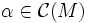$\alpha \in \mathcal{C}(M)$ now acts on$s$ the way it would originally have acted on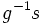$g^{-1}s$.

### Action with respect to the view of a connection as a splitting

Fill this in later

## Related facts

### The difference tensor

Given any$g \in G$, we can define the map: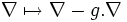$\nabla \mapsto \nabla - g.\nabla$

This difference is no longer a connection: in fact, since the connections form an affine space, the difference is a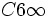$C6\infty$-bilinear map, or is a 2-tensor. This turns out to have important applications.

### The notion of a gauge

All connections that are in the same orbit under the action of the gauge group are essentially equivalent, so choosing a specific representative connection, is sometimes termed choosing a gauge, and is treated analogous to choosing a basis.

### The particular case of the tangent bundle

Further information: Gauge group of manifold acts on affine space of linear connections